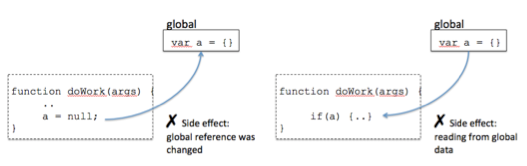//

# FUNCTIONAL PROGRAMMINGAbstract control flow and operations on data with functions in order to avoid side effects & reduce mutation of state

## Why Functional Programming?

Abstract control flow and operations on data with functions in order to:

• Avoid side effects
• Reduce mutation of state

## Pure Functions

Pure functions are different from impure functions because:

• It depends only on the input provided—and not on any hidden or external state that may change as a function’s evaluation proceeds or between function calls.
• It does not inflict changes beyond its scope (like modifying a global object or a parameter reference).### Referential Transparency

If a function consistently yields the same result on the same input, it is said to be `Referentially Transparent` (RT).

### Avoiding Loops

Pure functional programs don’t mutate variables (like a loop counter) after they’ve been initialized. So avoid while, and do-while. Instead, we will take advantage of high-order functions—like: forEach, map, reduce, and filter—to abstract iteration schemes, which also help us remove a few if-else conditionals while we’re at it.

### forEach

The forEach loop has the following advantages:

• Declarative and easier to understand
• Body being inside a function, it properly binds each value into the callback’s parameter.
• Because of No. 2, it avoids scoping bugs.
``````[80, 90, 100].forEach(function(value) {
console.log(value);
});
// 80, 90, 100
``````

### map

The map function has the following advantages:

• Transforms an array contents into an array of similar length as the original.
• Keeps the original array intact.
``````['a', 'b', 'c', 'd'].map(function(letter) {
return letter.toUpperCase();
});
// ["A", "B", "C", "D"]
``````

### filter

The filter function has the following advantages:

• Transforms an array by mapping a predicate function(a function with a boolean return value) on to each element.
• The returned array may contain a subset of the original values returned by the predicate.
``````['a', 'b', 'c', 'd'].map(function(letter) {
return letter.toUpperCase();
});
// ["A", "B", "C", "D"]
``````

### reduce

The reduce function has the following advantages:

Can be used to fold or gather the contents of an array by compressing it into a single value. Each successive invocation is supplied the return value of the previous. Seems a combination of map and filter.

``````[1, 2, 3, 4].reduce(function(total, num) {
});
// 10
``````

## Function Abstractions

Functional programming provides very powerful abstractions that allow you to create functions from the definition of other functions or to augment the evaluation of existing functions.

### currying

Currying is a technique that converts a multivariable function into a step-wise sequence of unary functions.

In other words, a function with parameters: f(a,b,c) is internally augmented to one that works on parameters one at a time: f(a) -> f(b) -> f(c)

``````var name = function(first) {
return function(last) {
console.log(first, last);
}
};
name(‘John’)(’Smith’);  // John Smith
``````

### Composition

Composition is the process used to group together the execution of simpler functions.

``````var compose = function () {
var fns = arguments;

return function (result) {
for (var i = fns.length - 1; i > -1; i--) {
result = fns[i].call(this, result);
}

return result;
};
};
var add = function(n) { return n + n; };
var multiply = function(n) { return n * n; };

``````

## Functional Data Types

Currying and composition provide abstractions over functions—the drivers for behavior in your application.

Functional data types like functors and monads provide abstractions over the data.

Functional data types part from the notion of mapping functions onto containers.

## Containerizing

The idea behind containerizing is very simple but has far-reaching applications. The goal is to place values in a container. By doing this, we hope to create a logical barrier that promotes transforming values immutably and side-effect free.

``````function getWords(val) {
return val.split(' ');
}

function getSize(array) {
return array.length;
}

function Wrapper(val) {
this.val = val;
}

Wrapper.prototype.map = function(f) {return f(this.val);};

var wrap = (val) => new Wrapper(val);

wrap('hello world').map(getWords).map(getSize); // [5, 5]
``````

In ES6, we can do the same like this:

``````const getWords = val => val.split(' ');

const getSize = array => array.length;

class Wrapper {
constructor(val) {
this.val = val;
}

map(f) {
return f(this.val);
}
}

var wrap = (val) => new Wrapper(val);

wrap('hello world').map(getWords).map(getSize);
``````

## Functors

The array map above is a functor since you can map a function onto its elements and the result is again an array.

A functor is nothing more than a type that implements a mapping function with the purpose of lifting values into a container.

``````F.prototype.map = function(f){

return F.of(f(this.val))
}
``````

The function `F.of` is a way to instantiate the data type without having to call new.

Functors are important because they introduce the idea of a function “reaching” into the container, modifying it, and placing the value “back into” it.